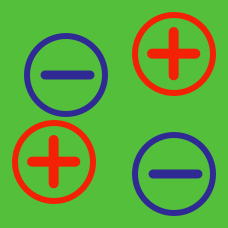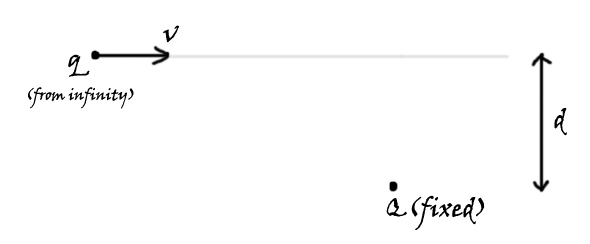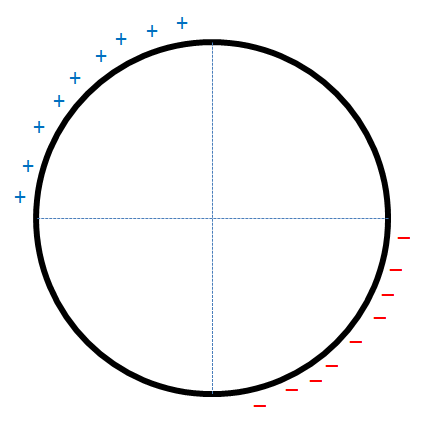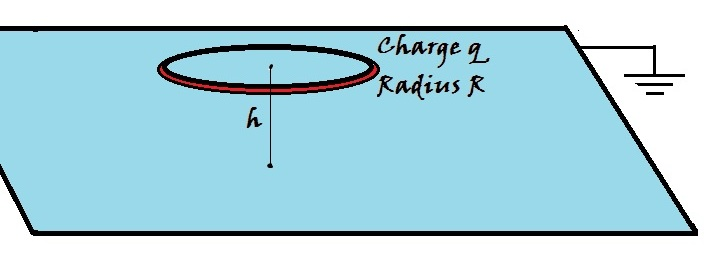Electricity and Magnetism

# Charges and Their Interactions: Level 3-4 Challenges

A particle of mass $m=1\text{ kg}$ and charge $q=3\text{ C}$ is hung from a light inextensible string of length $l=2\text{ m}$. The entire system is placed in a uniform horizontal electric field of magnitude $8\text{ V/m}$. What must be the minimum initial horizontal velocity with which the particle must be projected so that it completes a vertical circle?

Details and Assumptions

• The gravitational acceleration is $g=-10\text{ m/s}^2$.
• The particle is initially at the lowest point.

Consider two parallel, grounded, conducting plates located at $x=-L$ and $x=L$. A charge of $Q=-92 \space \mu\text{C}$ is placed at $(L/2,0,0)$. What is the total charge induced on the plate located at $x=L$ in microcoulombs?Let a charge $\displaystyle Q$ be held fixed in space. Another charge $\displaystyle q$ of mass $m$ is thrown with a velocity of $\displaystyle v$ from an infinitely far place, as shown in the figure, such that it is at a separation of $\displaystyle d$ initially.

Find the minimum distance (in m) between the two charges.

Details and Assumptions

$\bullet$ $\displaystyle Q = 5\ \mu C$
$\bullet$ $\displaystyle q = 1\ \mu C$
$\bullet$ $\displaystyle d = 3\text{ cm}$
$\bullet$ $\displaystyle v = 2 \text{ m/sec}$
$\bullet$ $\displaystyle m = 1\text{ g}$The figure shows a non-conducting, non-uniformly charged ring with linear charge densities $\lambda$ and $- \lambda$, mass $m$ and radius $R$. This ring is placed on a rough horizontal surface and a horizontal electric field $\vec{E}$ is switched on.

If at some instant, the ring is in the position shown above and is rolling without slipping, find the minimum coefficient of friction $\mu_{min}$ required.

Details & Assumptions

1. A uniform gravitational field $|\vec{g}| = 10 ms^{-2}$ acts downward.
2. $\lambda = \dfrac{\pi}{100} Cm^{-1}$
3. $m = 1.5 kg$
4. $R = 0.15 m$
5. $|\vec{E}| = 1000 NC^{-1}$A thin ring of radius $\displaystyle R$ is charged with a charge $\displaystyle q$. It is then placed over an infinitely large grounded conducting plane at a height $\displaystyle h$, as shown in the figure above.

Find the magnitude of the surface charge density $\displaystyle \sigma$ (in C/m$^2$) of the induced charge at the point on the plane which is exactly below the ring's center.

Details and Assumptions

$\bullet$ $\displaystyle q = 2\ \mu\text{C}$
$\bullet$ $\displaystyle R = 10\text{ cm}$
$\bullet$ $\displaystyle h = 15\text{ cm}$

×